Next: Traveling Salesman Problem Application Up: Methods Previous: Methods

## Circular tours

Definition 2.1   A Circular order C(T) of set of sequences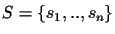is any tour through a tree T(S) where each edge is traversed exactly twice, and each leaf is visited once.

The problem can be solved by walking through the tree in a circular order, that is, from leaf A to B, from B to C, from C to D, from D to E and then back from E to leaf A (Figure 3), all edges are counted exactly twice, independent of the tree structure.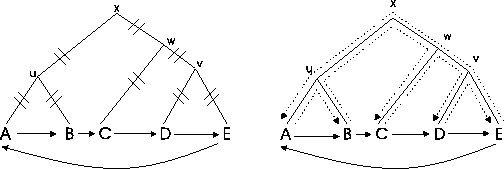Lemma 2.1 (Shortest Tour)   The circular tour is the shortest possible tour through a tree that visits each leaf once (see Figure 3). It traverses all edges exactly twice and thus weights all edges of the evolutionary tree equally.

Proof 2.1   Starting with 2 leaves, the proof is obvious: there is only one tour and all edges are counted exactly twice (see Figure 4).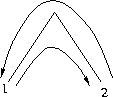When we have a tree with n leaves, subdivide the tree anywhere into the subtrees sA and sB (see Figure 5) and look at the edge x in the middle that connects the two subtrees. When the tree is traversed in the order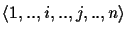and back to 1 in a circular tour, edge x is traversed exactly twice. Since the division into subtrees can be done anywhere in the tree, all edges are counted twice. There is no shorter tour, because we have to come back to the first leaf.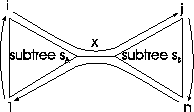Lemma 2.2 (Non-circular Tours)   Any other non-circular tour traverses at least one edge more than twice.

Proof 2.2   Again take a tree with n leaves, and subdivide the tree anywhere into the subtrees sA and sB (see Figure 6). When the tree is traversed in the order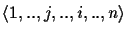and back to 1 (so we first go to j and then to i), edge x in the middle is traversed four times.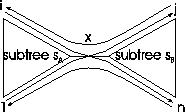For each tree T there exist 2(n-2) isomorphic forms. An isomorphic tree T' has the same tree topology as T, and it differs from T only in the sense that a subtree is rotated (in a graphical representation).

Lemma 2.3 (Isomorphic Trees)   A circular tour C(T) of a tree T is also a circular tour for all isomorphic trees of T.

Proof 2.3   Take the same tree as in Figure 5, but this time rotate one of the subtrees (leaves j and n are now on the opposite side as before)(see Figure 7). When the tree is traversed again in the orderand back to 1, the middle edge is only traversed twice. Again, since the division into subtrees can be done anywhere in the tree, all edges are counted twice, therefore this is true for all isomorphic trees.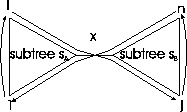An immediate conclusion from this is that any tree has 2(n-2) different circular tours which correspond to the circular orders of the isomorphic trees.

Definition 2.2   A tour Ci is shorter than a tour Cj (Ci < Cj) if the number of edges that are traversed by Ci is larger than the number of edges that are traversed by Cj.

Example 2.1:The order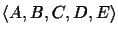is a circular order in Figure 8, but not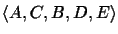. In the second example, edges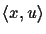and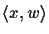are counted four times, while all other edges are counted only twice.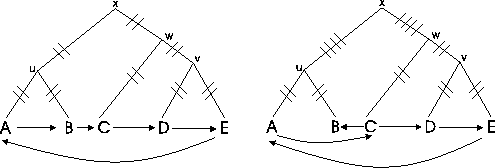Next: Traveling Salesman Problem Application Up: Methods Previous: Methods
Chantal Korostensky
1999-07-14